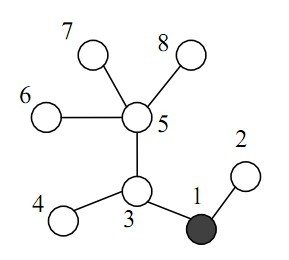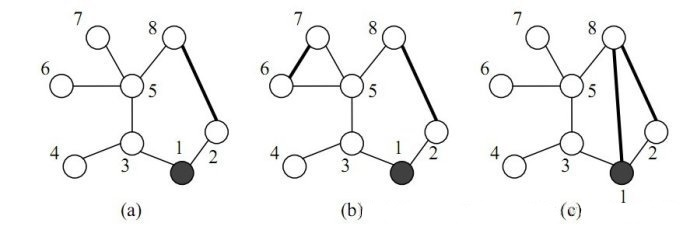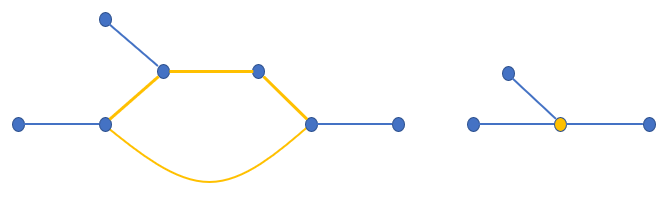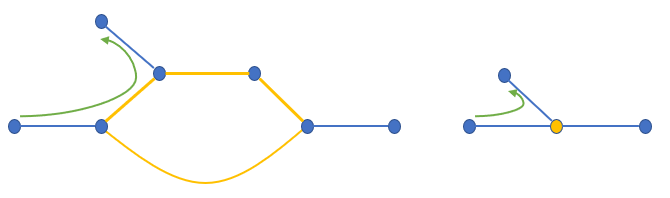# 洛谷 P3629 [APIO2010]巡逻 题解【树的直径】【树形DP】【贪心】

这个题真的只考树的直径啊。。。

## 题目描述8 1
1 2
3 1
3 4
5 3
7 5
8 5
5 6

11

8 2
1 2
3 1
3 4
5 3
7 5
8 5
5 6

10

5 2
1 2
2 3
3 4
4 5

6

## 说明

10%的数据中，n≤1000, K=1；

30%的数据中，K=1；

80%的数据中，每个村庄相邻的村庄数不超过 25；

90%的数据中，每个村庄相邻的村庄数不超过 150；

100%的数据中，3≤n≤100,000, 1≤K≤2。

我们如果要把每条道路都走一遍，根据树的性质，一定是每条边走2遍（隐含条件：回到1号点）。那么我们如果新建一条边，就会成环，如果链的长度为L，那么原来链上需要走的距离是L×2，现在变成了L+1，也就是只需要L+1次就可以把这个环走完并回到环的起点，所节省的距离为$2L-(L+1)=L-1$，随L，也就随链或环的大小单调递增。那么我们要使原来的链最长，就是树的直径。求树的直径有两种方法：两次dfs和树形DP。这道题方便起见，我两种都用到了。

首先当k=1时，求出直径，用所有边的2倍减去直径的长度再减1（意味着新加的一条边），就是答案。而当k=2时，我们要对这张图稍作处理。

首先根据贪心，我们需要做上一步的操作。对于下一步，我们要继续找树的直径。但是此时已经有一条链是被选入环里了，我的第一想法是把环缩掉，因为环不能对答案有贡献了，把环缩成一个点，这样就能找到新的直径，重复一开始的过程。但是这样是错的，因为环虽然不能对答案有正的贡献，但是会有负的。我们如果不管原来的环，只管没有缩掉的枝节，就会出现这种情况：如上是一个树的一部分，黄色部分是已经找出来的环。那么我们把它缩点成右边图的形式，缩点前后实际上是有区别的。左边原图的实际路程是3，而右侧只有2。而当k=1时，左边这条路径是5步，右边路径是4步。我们如果把这一段当成环，在右边的贡献是1（4-2[双向变单向]+1[新建的边]=3）。但看左边的图，中间一部分就重复走过了，贡献只有0（5-2[双向变单向]+1[单向变双向]+1[新建的边]=5）因此这种做法是错的。

通过研究这种错误的做法，发现环不能缩，而环上边的贡献是-1，我们就可以把原来直径上的边权（注意双向）改成-1，把这点负贡献归为直径长度的贡献，再做一遍第一步就可以了。

不过此时树上有负权边，两次DFS是个贪心（像dijkstra），不能处理负权边，我们要用树形DP求解；简单介绍一下树形DP方法：

Dfs(p->n);
mx=mx>f[x]+f[p->n]+p->v?mx:f[x]+f[p->n]+p->v;//先更新答案
f[x]=f[x]>f[p->n]+p->v?f[x]:f[p->n]+p->v;//再更新当前最优链

对于第一次找树的直径，并更新树的直径上的边权，用两次dfs做是最好的，因为没有负权，并且容易存储路径，也简单好写。

## Code：

#include<cstdio>
struct node
{
int n,v;
node *nxt,*op;
node(int n,int v)
{
this->n=n;
this->v=v;
nxt=NULL;
op=NULL;
}
node()
{
nxt=NULL;
op=NULL;
}
};
int d1,d2,L=0,l,flag=0;
int Pre;
node *pre;
void dfs(int x)
{
if(l>L)
{
L=l;
if(flag==0)
d1=x;
else
d2=x;
}
while(p->nxt!=NULL)
{
p=p->nxt;
if(p->n==Pre[x])
continue;
Pre[p->n]=x;
pre[p->n]=p;
l+=p->v;
dfs(p->n);
l-=p->v;
}
}
int f;
int mx=0;
void Dfs(int x)
{
f[x]=0;
int son=0;
while(p->nxt!=NULL)
{
p=p->nxt;
if(p->n==Pre[x])
continue;
Pre[p->n]=x;
Dfs(p->n);
mx=mx>f[x]+f[p->n]+p->v?mx:f[x]+f[p->n]+p->v;
f[x]=f[x]>f[p->n]+p->v?f[x]:f[p->n]+p->v;
}
}
int main()
{
int n,k,u,v;
scanf("%d%d",&n,&k);
for(int i=1;i<=n;i++)
for(int i=1;i<n;i++)
{
scanf("%d%d",&u,&v);
tail[u]->nxt=new node(v,1);
tail[u]=tail[u]->nxt;
tail[v]->nxt=new node(u,1);
tail[v]=tail[v]->nxt;
tail[u]->op=tail[v];//双向边都要修改
tail[v]->op=tail[u];
}
l=0;
Pre=1;//求路径用的
dfs(1);//求直径的一个端点
flag=1;
L--;
l=0;
Pre[d1]=d1;
dfs(d1);//求完整直径
flag=0;
int t=d2;
while(t!=d1)
{
pre[t]->v=-1;
pre[t]->op->v=-1;
t=Pre[t];
}
int ans=2*(n-1)-(L-1);//现在所要走的路程
if(k==1)
{
printf("%d\n",ans);
return 0;
}
Pre=1;
Dfs(1);//找新的直径
ans-=mx-1;
printf("%d\n",ans);
return 0;
}


### 2 说点什么0 Followers

Most reacted commentRecent comment authorswpDiscuz